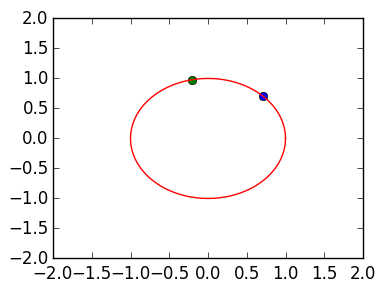# Permutation, orthogonal and triangular matrices¶

In this lecture we look at three types of matrices that are easy to invert: permutation matrices, orthogonal matrices and triangular matrices.

## Permutation matrices¶

Permutation matrices are matrices that represent the action of permuting the entries of a vector. As a very simple example, the following permutes the first and second entry:

In :
v=[1,2,3,4]
P12=[0 1 0 0;
1 0 0 0;
0 0 1 0;
0 0 0 1]
P12*v  # returns v with its first two entries swapped

Out:
4-element Array{Int64,1}:
2
1
3
4

Here are 2 more examples:

In :
v=[1,2,3,4]
P23=[1 0 0 0;
0 0 1 0;
0 1 0 0;
0 0 0 1]
P23*v  # returns v with its second and third entries swapped

Out:
4-element Array{Int64,1}:
1
3
2
4
In :
v=[1,2,3,4]
P312=[0 1 0 0;
0 0 1 0;
1 0 0 0;
0 0 0 1]
P312*v  # returns v with its first entry to sent to the 3rd entry,
# second entry sent to the first entry, and third entry sent to second entry

Out:
4-element Array{Int64,1}:
2
3
1
4

Permutations can be defined in Cauchy notation:

$$\begin{pmatrix} 1 & 2 & 3 & \cdots & n \cr \sigma_1 & \sigma_2 & \sigma_3 & \cdots & \sigma_n \end{pmatrix}$$

where $1,2,\ldots,n$ appear precisely once in the list $\sigma_1,\sigma_2,\ldots,\sigma_n$. This notation denotes the permutation that sends k to $\sigma_k$.

For example, the permutation

$$\begin{pmatrix} 1 & 2 & 3 & 4 \cr 4 & 3 & 1 & 2 \end{pmatrix}$$

sends the 1st entry to the fourth entry, second entry to third entry, third entry to first entry and fourthe entry to second entry.

We can convert this to matrix form:

In :
P=[0 0 1 0;
0 0 0 1;
0 1 0 0;
1 0 0 0]

Out:
4x4 Array{Int64,2}:
0  0  1  0
0  0  0  1
0  1  0  0
1  0  0  0

In general, the matrix corresponding to a permutation is given by

$$P = (\mathbf{e}_{\sigma_1} | \mathbf{e}_{\sigma_2} | \cdots | \mathbf{e}_{\sigma_n} )$$

The inverse of a permutation is the permutation that sends the entries back to where they came from. This is equivalent to swapping the rows in Cauchy's notation and sorting: the inverse of

$$\begin{pmatrix} 1 & 2 & 3 & 4 \cr 4 & 3 & 1 & 2 \end{pmatrix}$$

is the permutation

$$\begin{pmatrix} 1 & 2 & 3 & 4 \cr 3 & 4 & 2 & 1 \end{pmatrix}$$

This is precisely the transpose of $P$, and so we have the property that

$$P^\top P = I$$

for all permutation matrices.

In :
P'*P

Out:
4x4 Array{Int64,2}:
1  0  0  0
0  1  0  0
0  0  1  0
0  0  0  1

# Orthogonal matrices¶

A matrix is orthogonal if its inverse is its transpose:

$$Q^\top Q = I$$

Orthogonal matrices have the important property that they preserve the 2-norm of vectors:

$$\|Q\mathbf{v}\|^2 = (Q\mathbf{v})^\top Q \mathbf{v} = \mathbf{v}^\top Q^\top Q \mathbf{v} = \mathbf{v}^\top \mathbf{v} = \|\mathbf{v}\|^2$$

In 2D we get some simple examples:

$$\begin{pmatrix} 1 & 0 \cr 0 & 1\end{pmatrix}, \begin{pmatrix} 1 & 0 \cr 0 & -1\end{pmatrix}, \begin{pmatrix} 0 & 1 \cr 1 & 0\end{pmatrix}, \begin{pmatrix} \cos \theta & -\sin \theta \cr \sin \theta & \cos \theta \end{pmatrix}$$

The last matrix is a rotation matrix. We can visualize this using PyPlot, seeing that the 2-norm is preserved:

In :
using PyPlot

θ=1.     # rotation angle
Q=[cos(θ) -sin(θ);
sin(θ) cos(θ)]    # rotation matrix

v=[1,1]/sqrt(2)      # point on the circle
Qv=Q*v               # rotated vector
x=Qv     # x coordinate of the rotated vector
y=Qv     # y coordinate of the rotated vector

plot([v],[v];marker="o")    # plot the original point in blue
plot([x],[y];marker="o")         # plot the rotated point in green

# now plot the circle
grid=linspace(0,2π,100)       # plotting grid for the circle
plot(cos(grid),sin(grid))     # plot circle in red

axis((-2,2,-2,2))   # change viewport of the circleOut:
(-2,2,-2,2)

# Inverting an upper triangular matrix¶

We now discuss why upper triangular matrices are easy to invert. Consider a simple 3x3 example, which can be solved with \:

In :
U=[1.0 2.0 3.0;
0.0 3.0 4.0;
0.0 0.0 5.0]

b=[5,6,7]

x=U\b

Out:
3-element Array{Float64,1}:
0.533333
0.133333
1.4     
In :
norm(U*x -b)

Out:
8.881784197001252e-16

Behind the seens, \ is doing back substitution. Here is the 3x3 example done by hand:

In :
## my implementation \ for upper triangular matrices:

x=zeros(3)  # the solution vector

x=b/U[3,3]
x=(b-U[2,3]*x)/U[2,2]
x=(b-U[1,2]*x-U[1,3]*x)/U[1,1]

x  # same as U \b

Out:
3-element Array{Float64,1}:
0.533333
0.133333
1.4     

This algorithm is called back substitution. We can implement it for general dimensional matrices:

In :
function backsubstitution(U,b)
n=size(U,1)

if length(b) != n
error("The system is not compatible")
end

x=zeros(n)  # the solution vector
r=b[k]  # dummy variable
for j=k+1:n
r -= U[k,j]*x[j] # equivalent to r = r-U[k,j]*x[j]
end
x[k]=r/U[k,k]
end
x
end

Out:
backsubstitution (generic function with 1 method)
In :
backsubstitution(U,b)-U\b  # close to zero

Out:
3-element Array{Float64,1}:
5.55112e-16
3.05311e-16
-2.22045e-16

triu takes the upper triangular of a matrix. We can check the accuracy of backsubstitution on a 10 x 10 random upper triangular matrix.

In :
U=triu(rand(10,10))
b=rand(10)

x=U\b

norm(backsubstitution(U,b)-x)

Out:
8.545111909127925e-15